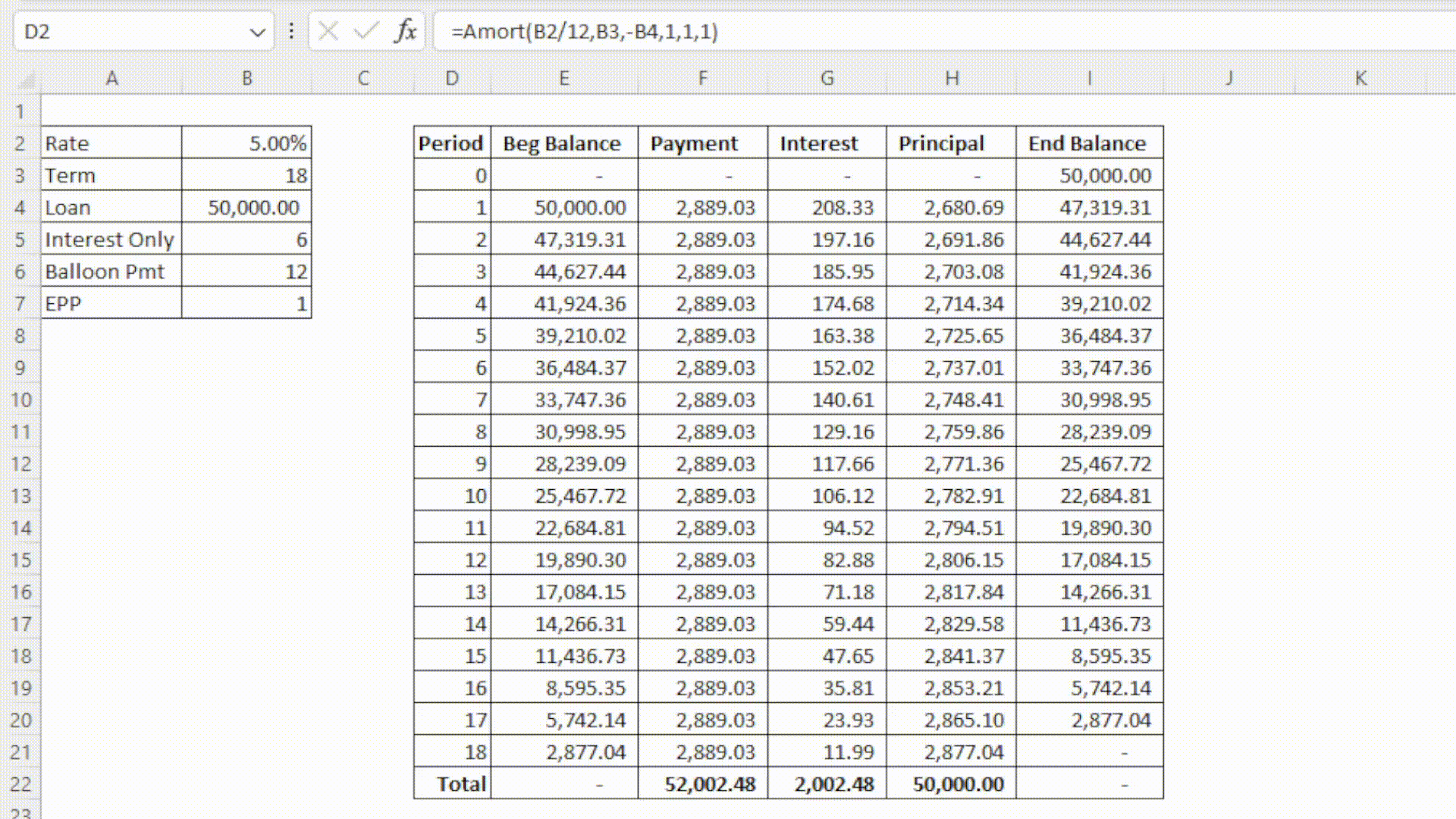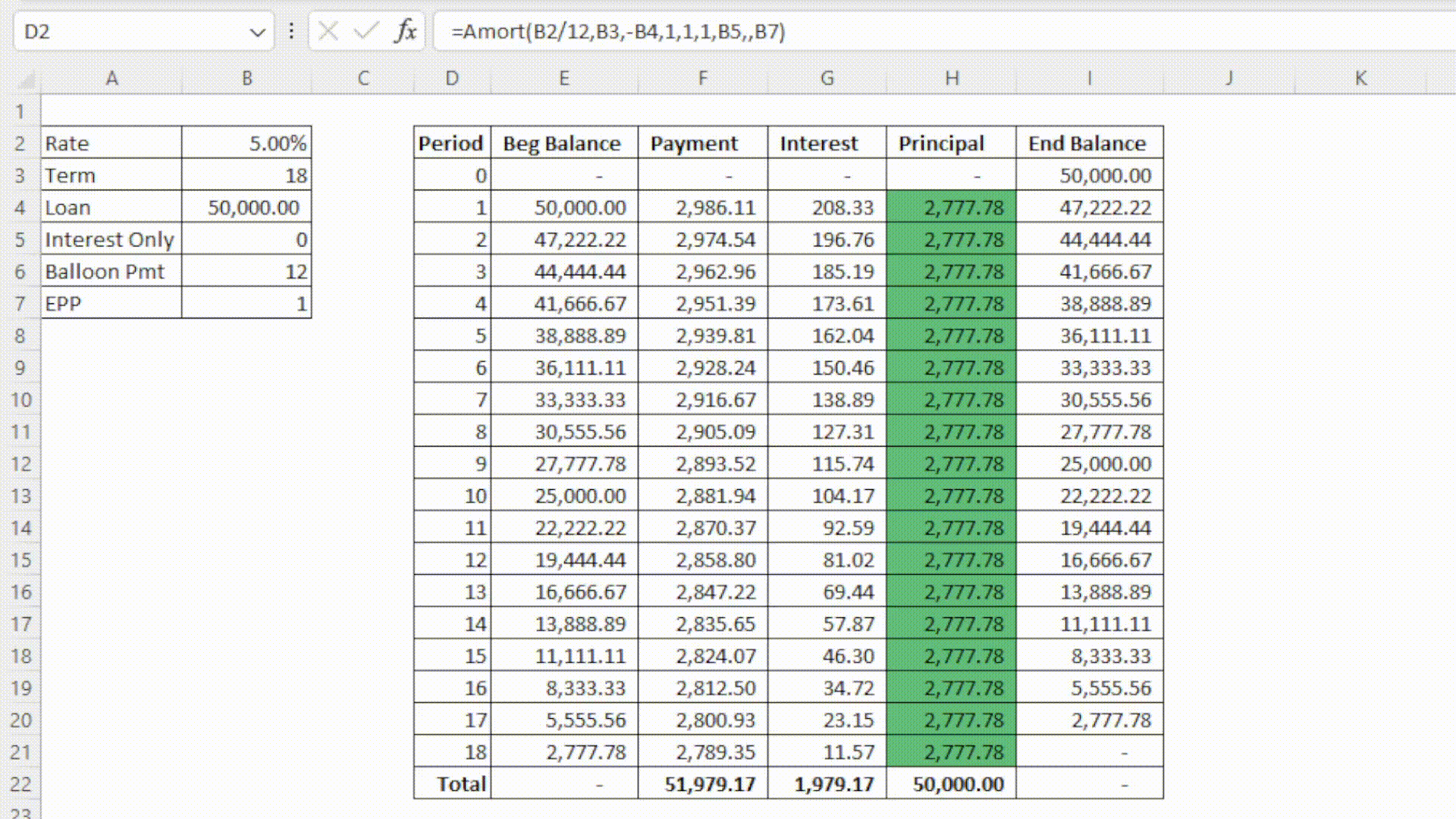top of page
• Nick

# Custom Amortization Function in Excel Using LAMBDA

Amort LAMBDA
.xlsx

The first ever financial model I can remember making was an amortization schedule, and I'd venture a guess that you've made one too. The set up and output always looks about the same:

1. Set your parameters in a snazzy looking bordered range in the top left of the sheet.

2. Set some simple titles for period number, beginning and ending balances, and payment information.

3. Get enough period numbers to cover the life of your loan.

4. Write the formulas for each column and drag down.

5. Pray to Satya Nadella that your ending balance at the last period is zero.

Like me, you've probably been doing this from scratch every time because you've gotten so used to the process, or maybe you have a template that you go back to each time and tweak it slightly. Since the advent of Array Formulas in Excel, you might have even simplified this process with SEQUENCE and other spilling formulas for different columns. Now with the release of the LAMBDA function in 2021 and LET in 2020, I've been starting to re-evaluate some processes that I use regularly to see just how useful some of these new functions can be.

Excel's new LAMBDA function allows user to take any function, set its inputs, and save that function to their workbook. I've recently used LAMBDA to create a new function, that I've called Amort, to take all the normal inputs of a amortization schedule model and spill out the entire results of the schedule. Here it is in action:Simple, right? Here's the code:

```=LAMBDA(rate,nper,pv,[headers],[totals],[roundOutput],[interestOnlyTerm],[balloonPmt],[EPP],
LET(
firstRow,HSTACK(SEQUENCE(1,5,0,0),-pv),
intOT,IF(ISOMITTED(interestOnlyTerm),0,interestOnlyTerm),
balloonPMT,IF(ISOMITTED(balloonPmt),nper,balloonPmt),
payoffTerm,MIN(nper,balloonPMT),
period,SEQUENCE(payoffTerm),
EPPPrincipal,SEQUENCE(payoffTerm,,IF(period<=intOT,0,-pv/(nper-intOT)),0),
cumEPPPrincipal,SCAN(0,EPPPrincipal,LAMBDA(a,i,a+i)),
stdPrincipal,IF(period<=intOT,0,PPMT(rate,period-intOT,nper-intOT,pv)),
cumStdPrincipal,SCAN(0,stdPrincipal,LAMBDA(a,i,a+i)),
balloonPayoff,IF(
period=balloonPMT,
-pv-CHOOSEROWS(IF(EPP=1,cumEPPPrincipal,cumStdPrincipal),balloonPMT-1),
0),
principal,IF(period=balloonPMT,balloonPayoff,IF(EPP=1,EPPPrincipal,stdPrincipal)),
endBalance,SCAN(-pv,principal,LAMBDA(a,i,a-i)),
begBal,VSTACK(-pv,DROP(endBalance,-1)),
interest,begBal*rate,
payment,interest+principal,
totalsRow,HSTACK(
"Total",
0,
ROUND(SUM(payment),2),
ROUND(SUM(interest),2),
ROUND(SUM(principal),2),
0),
initialData,VSTACK(firstRow,HSTACK(period,begBal,payment,interest,principal,endBalance)),
data,IF(roundOutput=1,ROUND(initialData,2),initialData),
dataWithTotals))

```

Ok, maybe not the simplest thing in the world. Don't worry if some of these functions are outside of your current Excel vocabulary, I'm going to break it down line by line. This may seem daunting, but I promise =IF(youCanPowerThroughTilEnd=TRUE,"You'll learn something new!","You'll be making amort tables from scratch forever.")

Note you'll need Excel 365 to use the function.

## Setting Up the Function

`=LAMBDA(rate,nper,pv,[headers],[totals],[roundOutput],[interestOnlyTerm],[balloonPmt],[EPP],`

The LAMBDA function always starts off with parameters. You can name as many parameters as you want 253 parameters, but we really only need a handful for our formula. You'll notice that there are brackets around the last 6 parameters. These are optional parameters that will add a some functionality and choices later. At the very least, the Amort function we're creating will require the input of rate, nper, and pv to spit out a vanilla amortization table. If any of these three inputs aren't provided, you'll get a VALUE! error. You'll see these required arguments as well as the optional arguments come back up as we move through the rest of the formula.

```LET(

The LET function is essential to building larger formulas like this. If you're not familiar with LET, it's a function that allows you to create names and assign values to those names that you can call back later within that same function. The entire remainder of this formula is wrapped up this one LET!

In this first LET parameter, we're simply defining a static row of titles by combining text in curly brackets separated by commas (using semicolons would create a column). We'll use these later if the user inputs a 1 for the [headers] parameter.

`     firstRow,HSTACK(SEQUENCE(1,5,0,0),-pv),`

Next we define the first line of our amortization table. Since this is period 0, with zeros in each column up until the ending balance of the new loan amount, we use SEQUENCE to spit out 5 horizontal zeros and use HSTACK to combine with our loan amount on the end. Note this pv is being passed from the LAMBDA function's input.

`     intOT,IF(ISOMITTED(interestOnlyTerm),0,interestOnlyTerm),`

An interest only term (shortened to intOT here) is a fairly common feature in some types of loans, and is included as an optional argument in this Amort function. If any integer is input to this field, the payments until that period will only cover interest, no principal payments will be made. We use the new ISOMITTED function here to check if this input was set: if it wasn't, we default to 0, if it was set then the input from the LAMBDA function is passed to the intOT parameter of LET.`     balloonPMT,IF(ISOMITTED(balloonPmt),nper,balloonPmt),`

Similar story here for the balloonPMT parameter. While not common in consumer lending, some term loans amortize over a period longer than the life of the loan, creating a large payment due at the end of the loan to pay the remaining principal. This is called a balloon payment or a bullet payment. This parameter is setting the period at which the balloon payment is due, if there is one, otherwise this is set to nper from the LAMBDA input.

```     payoffTerm,MIN(nper,balloonPMT),
period,SEQUENCE(payoffTerm),```

These next two lines are more straightforward, payoffTerm is just getting the term of the loan by finding the lower of the nper and balloonPMT, and period is creating an array of sequential numbers to label our period numbers. Note that period starts at 1 but the final output starts at 0, can you figure out why?

## Core of the Amortization Table

```     stdPrincipal,IF(period<=intOT,0,PPMT(rate,period-intOT,nper-intOT,pv)),
cumStdPrincipal,SCAN(0,stdPrincipal,LAMBDA(a,i,a+i)),```

Now we get into the meat of the output. In this stdPrincipal (standard principal) parameter, we will be setting the entire loan's principal payments from the first period to the last. We first use IF to check if we're still in the interest only period, if we are then our principal payment will be 0. Otherwise, we use the PPMT function to calculate our principal payment based on the period. PPMT is very similar to PMT; it returns the principal payment portion of a payment based on what period you're at in the amortization. Since we're pointing the the per option of PPMT at an array of periods, this will return an array of the principal payments for each period.

In cumStdPrincipal (cumulative standard principal), we're using the new Excel LAMBDA helper function SCAN to get a running total by period. This will be used for the balloon payment option. This could also be achieved with the CUMPRINC function, but I couldn't pass up a perfectly good opportunity to use the new function.

SCAN may look complicated, but in it's simplest form this is all there is to it is:

1. First argument is the starting point (0)

2. Second argument is an array (our principal payments)

3. Third you enter a LAMBDA function, followed by two parameters (named whatever you want, but they correspond to arguments 1 and 2 of SCAN).

4. The calculation portion of LAMBDA is how you want to accumulate. In this case, we're just adding up principal in each period to get a running total of principal paid.

```     EPPPrincipal,SEQUENCE(payoffTerm,,IF(period<=intOT,0,-pv/(nper-intOT)),0),
cumEPPPrincipal,SCAN(0,EPPPrincipal,LAMBDA(a,i,a+i)),```

The last major optional feature of Amort is for equal principal payments (EPP). I've never actually seen a loan structured like this, but anything is possible in the magical land of finance, and from what I understand this is more popular in markets outside the US. An EPP loan's payment goes down over time, while the principal portion of the loan is flat over the term. This is contrary to the normal amortization when a payment is flat over the term of the loan and the principal portion increases as the interest portion of your payment decreases over time.

These two parameters are set up in a similar way to the standard principal. The big difference is that we can take advantage of SEQUENCE to spit of the same number for each period of the loan, cause you know, equal principal payments. Note that we are dividing the pv (loan amount) by the nper (term of loan) minus the intOT to compensate for a potential interest only period.```balloonPayoff,IF(period=balloonPMT,
-pv-CHOOSEROWS(IF(EPP=1,cumEPPPrincipal,cumStdPrincipal),balloonPMT-1),0),```

Here we are setting a parameter equal to the amount that will need to be paid off (remaining balance of loan) at the balloon payment period (normal principal if there is no balloon payment). To do this, we take the loan amount and subtract total principal paid up until the period before the balloon payment. The cumulative principal at the period before the balloon payoff comes from the CHOOSEROWS function.`    principal,IF(period=balloonPMT,balloonPayoff,IF(EPP=1,EPPPrincipal,stdPrincipal)),`

Now that we've done most of the heavy lifting, these last few lines will come a little easier. This principal parameter is just an IF exercise of combining what we've already put together to get a final principal column.

```     endBalance,SCAN(-pv,principal,LAMBDA(a,i,a-i)),
begBal,VSTACK(-pv,DROP(endBalance,-1)),```

To get the ending balance of each period, we'll use the SCAN function again. The starting value of ending balance is going to be the total loan value (-pv), and we'll decrease that amount by the principal payment each period.

Though not necessary to set up this way, I've made begBalance dependent on endBalance to ensure the ending balance transfers directly to the next period's beginning balance. VSTACK here is creating a column that starts with -pv and appends on all but the last row of ending balance, brought to you by the new DROP function.

```          interest,begBal*rate,
payment,interest+principal,```

The interest and payment parameters are just an exercise of arithmetic now that we have our other columns made. Note: interest is multiplying begBal by the rate, set directly in the LAMBDA function.

```          totalsRow,HSTACK(
"Total",
0,
ROUND(SUM(payment),2),
ROUND(SUM(interest),2),
ROUND(SUM(principal),2),
0),```

Hang in there, this is the last real parameter before we start compiling for the output. totalsRow here is just creating a row with HSTACK that we'll tack on to the bottom of the amort range if the user chooses that option. The ROUND functions here aren't necessary, just my preference.

## Putting it All Together

```          initialData,VSTACK(firstRow,HSTACK(period,begBal,payment,interest,principal,endBalance)),
data,IF(roundOutput=1,ROUND(initialData,2),initialData),
dataWithTotals))```

Now to compile our work. This is done in a few steps really just for organization.

1. initialData: Using H/VSTACK to compile together just the numbers of our output, no headers or totals row.

2. data: If the user has opted to round each number in the table, they'll be rounded here. Note that this does not affect the totals row or the interest calculations since it's happening after these have all been set.

4. dataWithTotals: VSTACKing on the total row if chosen in Amort.

At the very end of the function, we simply return dataWithTotals to spit out our amortization table!

I hope you found this useful and learned something new! Please let me know what you found most useful or something you didn't understand in the comment section below.

To be notified of any future posts, please be sure to subscribe below!

-Nick